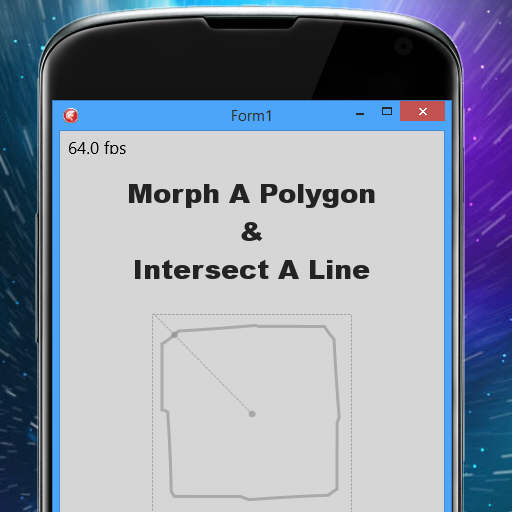Get access to over 100 FireMonkey cross platform samples for Android, IOS, OSX, Windows, and Linux!

# Polygon Morphing And Line Intersection With Delphi XE6 Firemonkey On Windows And OSXDeveloper zedalaye has a project up on Github which demonstrates how to intersect a polygon that is morphing in real time with a line. The original project was for Delphi XE2 but I have updated the project for Delphi XE6 Firemonkey. I was able to get it working on Windows in XE6 but under Android there is a painting issue. Basically it has a polygon shape in the center of a TPaintBox. There is a line that rotates from the center to the edges of the box and wherever the line intersects with the polygon a point is drawn. At the same time the polygon is morphing it’s shape so the point where the line is intersecting the polygon is constantly changing. There is some interesting code in here including two custom classes that derive from the TAnimation component for handling the animation of the point and the polygon. Take a look at the two objects here:
```TPointAnimation = class(TAnimation) private type TBorder = (bTop, bRight, bBottom, bLeft); private var FBorder: TBorder; FControl: TControl; FEndSegment: TPointF; FFirstPass: Boolean; protected procedure ProcessAnimation; override; public constructor Create(AOwner: TComponent); override; property Control: TControl read FControl write FControl; property Point: TPointF read FEndSegment; end;```

``` ```

```TPolygonAnimation = class(TAnimation) private FControl: TControl; FStock: array of TPolygon; FBase, FTarget: Integer; FShape: TPolygon; procedure SetControl(const Value: TControl); procedure ResamplePolygon(Index: Integer; FinalPoints: Integer); protected procedure ProcessAnimation; override; procedure Start; override; public constructor Create(AOwner: TComponent); override; property Control: TControl read FControl write SetControl; property Shape: TPolygon read FShape; end;```
And here is the intersect function which will give you the TPointF where two lines intersect.
`function Intersect(const A1, A2, B1, B2: TPointF; out P: TPointF): Boolean;`

``` { Returns the determinant } function Det(P1, P2: TPointF): Single; inline; begin Result := P1.X * P2.Y - P1.Y * P2.X; end; { Returns True if Min(X1, X2) <= X < Max(X1, X2) } function InSignedRange(const X, X1, X2: Single): Boolean; inline; begin Result := (X < X1) xor (X < X2); end; var A, B, AB: TPointF; dAB, dBAB, dAAB: Single; begin Result := False; A := A2 - A1; B := B2 - B1; dAB := Det(A, B); if dAB = 0 then Exit; { vectors A and B hold by (A1,A2) and (B1,B2) are colinear } AB := A1 - B1; dAAB := Det(A, AB); dBAB := Det(B, AB); ```

```if InSignedRange(dAAB, 0, dAB) and InSignedRange(dBAB, 0, dAB) then begin Result := True; dBAB := dBAB / dAB; P.X := A1.X + dBAB * A.X; P.Y := A1.Y + dBAB * A.Y; end; end;```
Head over and check out the source code for the polygon morphing and line intersection project on Github.

Or download the updated polygon morphing and line intersection project for Delphi XE6 Firemonkey.